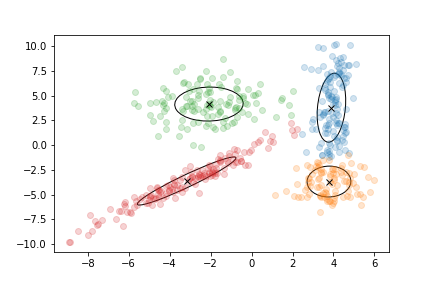Getting started¶

Installation¶

The package is registered on PyPI. It can be installed with the following command:

\$ pip install megamix

If you want to install it manually, you can find the source code at https://github.com/14thibea/megamix.

MeGaMix relies on external dependencies. The setup script should install them automatically, but you may want to install them manually. The required packages are:

• cython

Note

If you cannot compile the package, please dowload it and manually comment the line ext_modules=cythonize(ext_modules), in setup.py Doing so you will not compile the cython modules and only use pure python versions.

Description¶

The MeGaMix package (Methods for Gaussian Mixtures) allows Python developpers to fit different kind of models on their data. The different models are clustering methods of unsupervised machine learning. Four models have been implemented, from the most simple to the most complex:

• K-means
• GMM (Gaussian Mixture Model)
• VBGMM (Variational Bayesian Gaussian Mixture Model)
• DP-VBGMM (Dirichlet Process on Variational Bayesian Gaussian Mixture Model)
• PYP-VBGMM (Pitman-Yor Process on Variational Bayesian Gaussian Mixture Model)

What will you be able to do ?¶

The main idea of clustering algorithms is to create groups by gathering points that are close to each other.

A cluster has three main parameters:

• A mean : the mean of all the points that belong to the cluster
• A weight : the number of points that belong to the cluster
• A covariance (except for K-means) : a matrix which specifies the form of the clusterA graphical example of a gaussian mixture model fit on a set of points

How do the algorithms work ?¶

After the initialisation, the algorithms alternate between two steps, the E step (Expectation) and the M step (Maximisation).

During the E step, the algorithm computes the probability for each point to belong to each cluster. It produces an array of responsibilities. At the ith row and the jth column of this array corresponds the probability of the ith point to belong to the jth cluster.

Here is an example of responsibilities that could be obtained with 6 points and 2 clusters :

Cluster 1 Cluster 2
point 1 0.54 0.46
point 2 0.89 0.11
point 3 0.27 0.73
point 4 0.01 0.99
point 5 0.42 0.58
point 6 0.84 0.16

In this example, the first point has a 54% chance to belong to the first cluster and a 46% chance to belong to the second cluster.

Note

This is not the case with K-means which is not working with probabilities but with labels. A point belongs completely to a cluster or doesn’t belong to it (this is called hard assignement).

Then during the M step, the algorithm re-estimates the parameters of the model in order to maximize a convergence criterion.

Finally the algorithm will stop if the difference between the value of the convergence criterion of the current and the previous is less than a threshold fixed by the user.

This is summarized in the following pseudo-code:

initialize(points)
while(cc-cc_previous > tol):
cc_previous = cc
responsabilities = E_step(points,parameters)
parameters = M_step(responsabilities,points)
cc = convergence_criterion(points,responsabilities,parameters)

What is it used for ?¶

MeGaMix has been implemented in order to process natural speech MFCC. Unlike the vision field where deep learning has overtaken such clustering models, they are still efficient in speech processing.

However the use of this package is more general and may serve another purpose.

Basic usage¶

########################
# Prelude to the example
########################
"""
This example is realized with a DP-VBGMM model
The other mixtures and the K-means are working in the same way
The available classes are:
- Kmeans (kmeans)
- GaussianMixture (GMM)
- VariationalGaussianMixture (VBGMM)
- DPVariationalGaussianMixture (DP-VBGMM)
"""

from megamix import DPVariationalGaussianMixture
import numpy as np

########################
# Features used
########################

"""
Features must be numpy arrays of two dimensions:
the first dimension is the number of points
the second dimension is the dimension of the space
"""

# Here we use a radom set of points for the example
n_points = 10000
dim = 39

points = np.random.randn(n_points,dim)

########################
# Fitting the model
########################

# We choose the number of clusters that we want
n_components = 100

# The model is instantiated
GM = DPVariationalGaussianMixture(n_components)

# The model is fitting
GM.fit(points)

# It is also possible to do early stopping in order to avoid overfitting
points_data = points[:n_points//2:]
points_test = points[n_points//2::]

# In this case the model will fit only on points_data but will use points_test
# to evaluate the convergence criterion.
GM.fit(points_data,points_test)

# Some clusters may disappear with the DP-VBGMM model. You may want to
# simplify the model by removing the useless information
GM_simple = GM.simplified_model(points)

##########################
# Analysis of the model
##########################

other_points = np.random.randn(n_points,dim)

# We can obtain the log of the reponsibilities of any set of points when the
# model is fitted (or at least initialized)
log_resp = GM.predict_log_resp(other_points)
# log_resp.shape = (n_points,n_components)

# We can obtain the value of the convergence criterion for any set of points
score = GM.score(other_points)

#############################
# Writing or reading a model
#############################

# It is possible to write your model in a group of a h5py file
import h5py

file = h5py.File('DP_VBGMM.h5','w')
grp = file.create_group('model_fitted')

GM.write(grp)
file.close()

# You also can read data from such h5py file to initialize new models
GM_new = DPVariationalGaussianMixture()

file = h5py.File('DP_VBGMM.h5','r')
grp = file['model_fitted']